Courses

# NCERT Exemplar - Atoms and Molecules (part-3) Class 9 Notes | EduRev

## Class 9 : NCERT Exemplar - Atoms and Molecules (part-3) Class 9 Notes | EduRev

The document NCERT Exemplar - Atoms and Molecules (part-3) Class 9 Notes | EduRev is a part of the Class 9 Course Science Class 9.
All you need of Class 9 at this link: Class 9

Q.29. A gold sample contains 90% of gold and the rest copper. How many atoms of gold are present in one gram of this sample of gold?
Ans.
Amount of gold in 1 g of 90% pure gold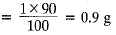1 mole of Au = 197 g
1 mole of Au = 6.022 x 1023 atoms
197 g of gold contains 6.022 x 1023 atoms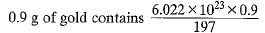= 2.75 x 1021 atoms

Q.30. What are ionic and molecular compounds? Give examples.
Ans.
Ionic compounds are those compounds which are solid and form ions in aqueous solution, have high melting and boiling points, do not conduct electricity in solid state but conduct electricity in molten state or in aqueous solution, e.g. NaCl, KCl, MgO, CaO, etc Molecular compounds may be solids, liquids or gases, do not form ions in aqueous solution, have low melting and boiling points, do not conduct electricity e.g. CH4, CCl4, NH3, PH3, etc.

Q.31. Compute the difference in masses of one mole each of aluminium atoms and one mole of its ions. (Mass of an electron is 9.1 x 10-28 g).
Which one is heavier?
Ans.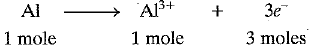Mass of 1 electron = 9.1 x 10-28 g
Mass of 3 x 6.022 x 1023 electrons
= 9.1 x 10-28 x 3 x 6.022 x 1023
Mass of 3 moles of electrons = 164.400 x 10-5 g
= 0.00164 g
Molar mass of Al3+ ions = 27 - 0.00164 g
= 26.9984 g mol-1
Difference in mass between Al and Al3+=0.00164 g
Al atom is heavier than Al3+.

Q.32. A silver ornament of mass ‘m’ gram is polished with gold equivalent to 1% of the mass of silver. Compute the ratio of the number of atoms of gold and silver in the ornament.
Ans.
Mass of silver in the ornament = m gram
Mass of gold in the ornament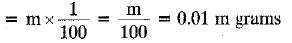108 g of Ag contains 6,022 x 1023 atoms
m gram of Ag contain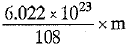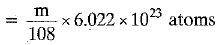197 g of Au contains 6.022 x 1023 atoms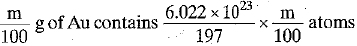Ratio of number of atoms of gold and silver
= Au : Ag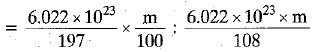= 108 : 19700 = 1 : 182.41

Q.33. A sample of ethane (C2H6) gas has the same mass as 1.5 x 1020 molecules of methane (CH4). How many C2H6 molecules does the sample of gas contain?
Ans.
1 mole of CH4 = 16 g
1 mole of CH4 contains 6.022 x 1023 molecules 6.022 x 1023 molecules of CH4 has mass = 16 g
1.5 x 1020 molecules of CH4 has mass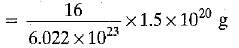Now, 1 mole of C2H6 = 30 g
1 mole of C2H6 = 6.022 x 1023 molecules
30 g of C2H6 contains 6.022 x 1023 moles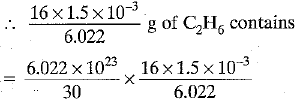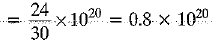= 8 x 1019 molecules

Q.34. Fill in the blanks:
(a) In a chemical reaction, the sum of the masses of the reactants and products remains unchanged. This is called_______.
(b) A group of atoms carrying a fixed charge on them is called_______.
(c) The formula unit m ass of Ca3(PO4)2 is _______ . [Ca = 40 u, P = 31 u, O = 16 u]
(d) Formula of sodium carbonate is _______. and that of ammonium sulphate is______.

Ans. (a) Law of conservation of mass
(c) 40 x 3 + 2 x 31 + 8 x 16
= 120 + 62 + 128 = 310 u
(d) Na2CO3; (NH4)2SO

Q.35. Complete the following crossword puzzle by using the name of the chemical elements. Use the data given in Table.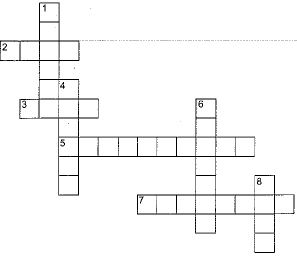Across
2. The element used by Rutherford during his a-scattering experiment (4)
3. An element which forms rust on exposure to moist air (4)
5. A very reactive non-metal stored under water (10)
7. Zinc metal, when treated with dilute hydrochloric acid, produces a gas of this element which when tested with burning splinter produces a pop sound. (8)
Down
1. A white lustrous metal used for making ornaments and which tends to get tarnished black in the presence of moisture (6)
4. Both brass and bronze are alloys of the element (6)
6. The metal which exists in the liquid state at room temperature (7)
8. An element with symbol Pb (4)
Ans.
> Across :
2. GOLD
3. IRON
5. PHOSPHORUS
7. HYDROGEN
> Down :
1. SILVER
4. COPPER
6. MERCURY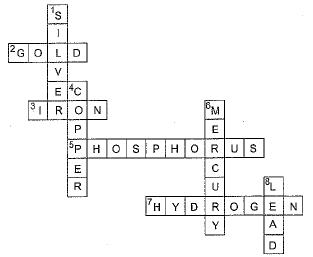Q.36. (a) In the given crossword puzzle, names of 11 elements are hidden. The symbols of these elements are given below. Complete the puzzle.
1. C
2. H
3. Ar
4. O
5. Xe
6. N
7. He
8. F
9. Kr
10. Rn
11. Ne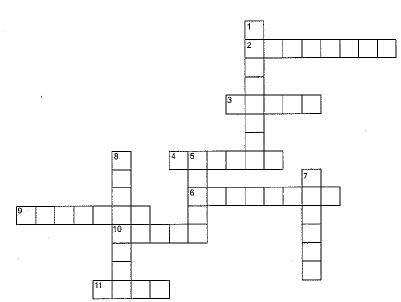(b) Identify the total number of inert gases, their names and symbols from this crossword puzzle.
Ans.
(a)
1. Cl-CHLORINE
2. H-HYDROGEN
3. Ar-ARGON
4. O-OXYGEN
5. Xe-XENON
6. N-NITROGEN
7. He-HELIUM
8. F-FLUORINE
9. Kr-KRYPTON
11. Ne-NEON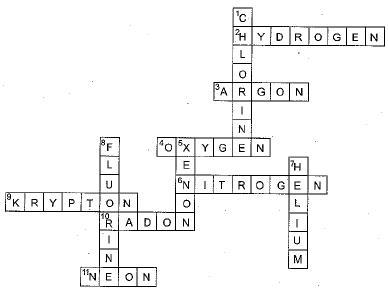(b) Helium (He), Neon (Ne), Argon (Ar), Krypton (Kr), Xenon (Xe) and Radon (Rn) are six inert gases.

Q.37. Write the formulae for the following and calculate the molecular mass for each one of them.
(a) Caustic potash
(b) Baking powder
(c) Lime stone
(d) Caustic soda
(e) Ethanol
(f) Common salt
Ans.
(a) Caustic potash is KOH, Molecular mass
= 39 + 16 + 1 = 56 u
(b) Baking powder is NaHC03, Molecular mass
= 23 + 1 + 12 + 48 - 84 u
(c) Lime stone is CaC03, Molecular mass
= 40 + 12 + 48 - 100 u
(d) Caustic soda is NaOH, Molecular mass
= 23 + 16 + 1 = 40 u
(e) Ethanol is C2H5OH, Molecular mass
= 24 + 5 + 16 + 1 = 46 u
(f) Common salt is NaCl, Molecular mass
= 23 + 35.5 = 58.5 u

Q.38. In photosynthesis, 6 molecules of carbon dioxide combine with an equal number of water molecules through a complex series of reactions to give a molecule of glucose having a molecular formula C6H12O6. How many grams of water would be required to produce 18 g of glucose? Compute the volume of water so consumed, assuming the density of water to be 1 g cm-3.
Ans.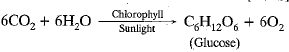Molar mass of C6H12O
= 6 x 12 + 12 x 1 + 6 x 16
= 72 + 12 + 96 = 180 g mol-1
180 g of C6H12O6 needs 108 g of H20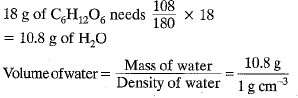Volume of water = 10.8 cm3

Offer running on EduRev: Apply code STAYHOME200 to get INR 200 off on our premium plan EduRev Infinity!

## Science Class 9

143 videos|224 docs|135 tests

,

,

,

,

,

,

,

,

,

,

,

,

,

,

,

,

,

,

,

,

,

;